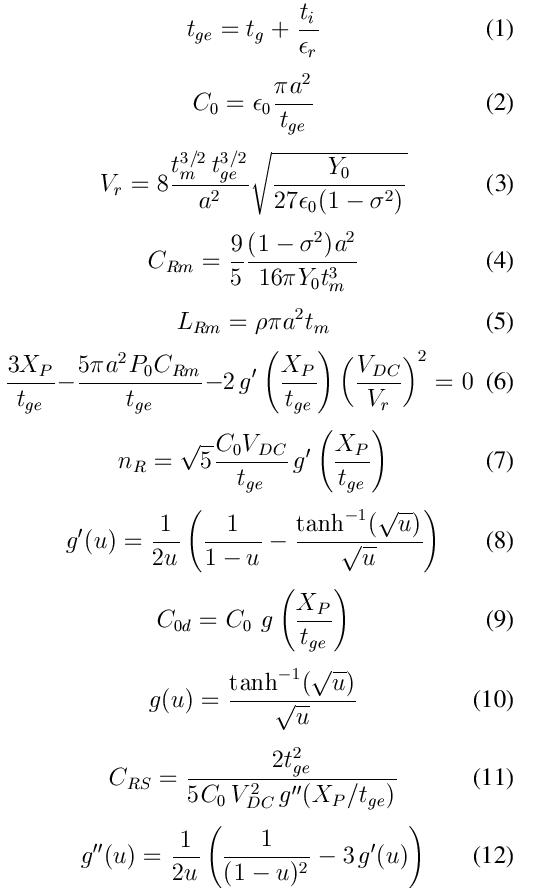The small-signal equivalent circuit of an uncollapsed CMUT cell is given in the figure.  The parameters on the acoustic side are dependent on the lumped variable used to represent the velocity or force in the acoustic side. The equivalent circuit shows the parameters with rms variables. The velocity of the top plate is represented by an spatial rms velocity averaged over the area of the top plate (current in the acoustic side).

Parameters of the circular CMUT cell (all in MKS units)

• a : Radius of the cell
• tg : gap height of the cell
• ti : thickness of the insulator
• tm : thickness of the top plate
• εr : relative permitivity of the insulator
• P0 : Ambient pressure
• Y0 : Young’s modulus of the top plate
• σ : Poisson’s ratio of the top plate
• ρ : density of the top plate
• VDC: DC bias voltage at the electrical portParameters in the equivalent circuit may be found using the equations given below.

• tge is the effective gap height: Eq (1)
• Vr is the collapse voltage in the absence of ambient pressure: Eq (3)
• CRm represents the compliance of the top plate: Eq (4)
• LRm is due to mass of the top plate: Eq (5)
• XP is the deflection of the top plate at its center: Eq (6)
• nR is the turns ratio of electromechanical transformer: Eq (7)
• C0d is the input electrical capacitance including the effect of top plate deflection: Eq (9)
• CRS arises from the spring-softening effect: Eq (11)

Refer to the paper by Koymen, et.al for details.